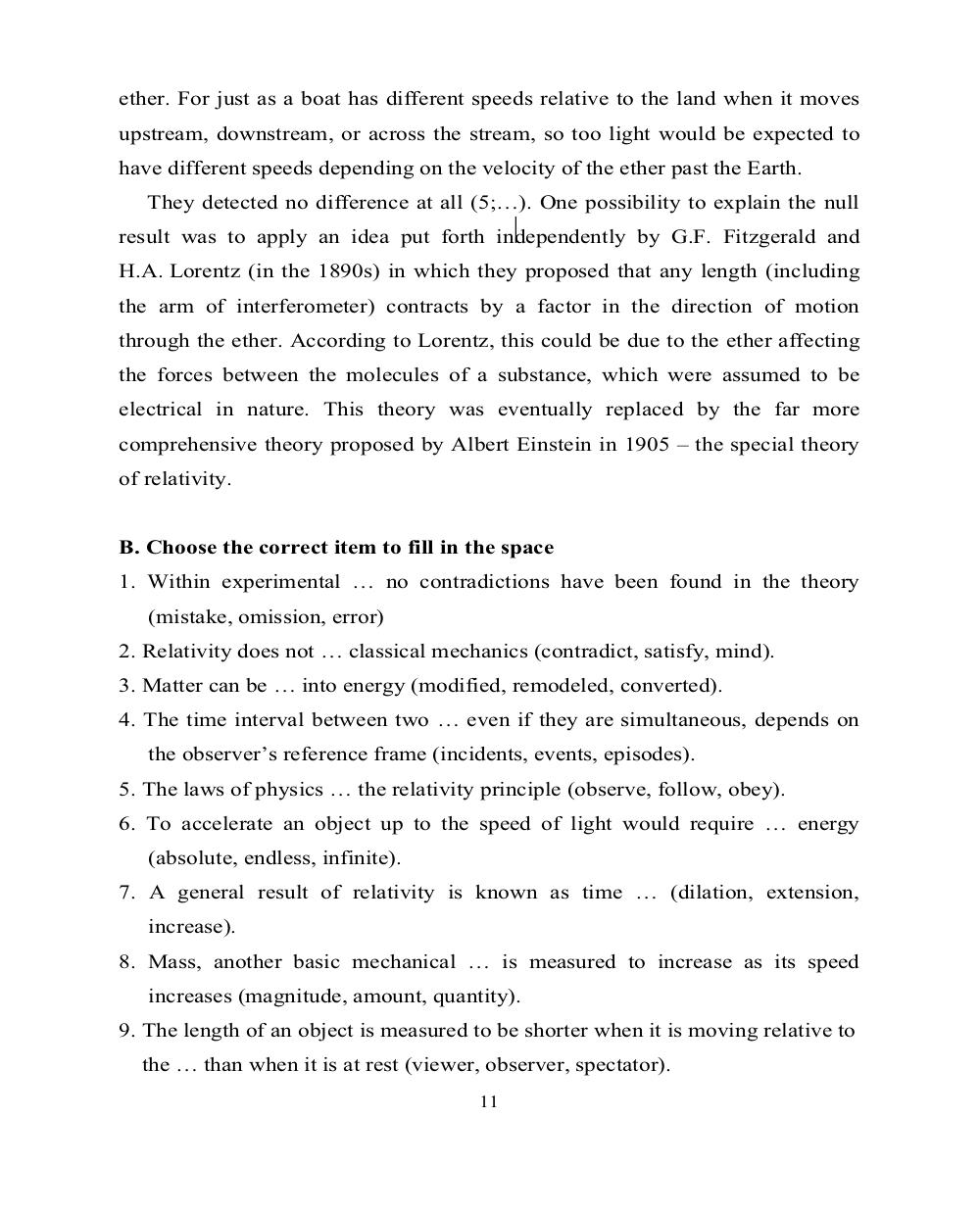# Английский язык. Ч.3 (Tests 10,11,12). Ильичева Н.А - 11 стр.

Составители:

Рубрика:

• ## Иностранный языкether. For just as a boat has different speeds relative to the land when it moves
upstream, downstream, or across the stream, so too light would be expected to
have different speeds depending on the velocity of the ether past the Earth.
They detected no difference at all (5;…). One possibility to explain the null
result was to apply an idea put forth independently by G.F. Fitzgerald and
H.A. Lorentz (in the 1890s) in which they proposed that any length (including
the arm of interferometer) contracts by a factor in the direction of motion
through the ether. According to Lorentz, this could be due to the ether affecting
the forces between the molecules of a substance, which were assumed to be
electrical in nature. This theory was eventually replaced by the far more
comprehensive theory proposed by Albert Einstein in 1905 – the special theory
of relativity.
B. Choose the correct item to fill in the space
1. Within experimental … no contradictions have been found in the theory
(mistake, omission, error)
2. Relativity does not … classical mechanics (contradict, satisfy, mind).
3. Matter can be … into energy (modified, remodeled, converted).
4. The time interval between two … even if they are simultaneous, depends on
the observer’s reference frame (incidents, events, episodes).
5. The laws of physics … the relativity principle (observe, follow, obey).
6. To accelerate an object up to the speed of light would require … energy
(absolute, endless, infinite).
7. A general result of relativity is known as time … (dilation, extension,
increase).
8. Mass, another basic mechanical … is measured to increase as its speed
increases (magnitude, amount, quantity).
9. The length of an object is measured to be shorter when it is moving relative to
the … than when it is at rest (viewer, observer, spectator).
11ether. For just as a boat has different speeds relative to the land when it moves
upstream, downstream, or across the stream, so too light would be expected to
have different speeds depending on the velocity of the ether past the Earth.
They detected no difference at all (5;…). One possibility to explain the null
result was to apply an idea put forth independently by G.F. Fitzgerald and
H.A. Lorentz (in the 1890s) in which they proposed that any length (including
the arm of interferometer) contracts by a factor in the direction of motion
through the ether. According to Lorentz, this could be due to the ether affecting
the forces between the molecules of a substance, which were assumed to be
electrical in nature. This theory was eventually replaced by the far more
comprehensive theory proposed by Albert Einstein in 1905 – the special theory
of relativity.

B. Choose the correct item to fill in the space
1. Within experimental … no contradictions have been found in the theory
(mistake, omission, error)
2. Relativity does not … classical mechanics (contradict, satisfy, mind).
3. Matter can be … into energy (modified, remodeled, converted).
4. The time interval between two … even if they are simultaneous, depends on
the observer’s reference frame (incidents, events, episodes).
5. The laws of physics … the relativity principle (observe, follow, obey).
6. To accelerate an object up to the speed of light would require … energy
(absolute, endless, infinite).
7. A general result of relativity is known as time … (dilation, extension,
increase).
8. Mass, another basic mechanical … is measured to increase as its speed
increases (magnitude, amount, quantity).
9. The length of an object is measured to be shorter when it is moving relative to
the … than when it is at rest (viewer, observer, spectator).
11